Civil Engineering - Applied Mechanics

Exercise :: Applied Mechanics - Section 3

36.

A trolley wire weighs 1 kg per metre length. The ends of the wire are attached to two poles 20 m apart. If the horizontal tension is 1000 kg, the central dip of the cable is

 A. 2 cm B. 3 cm C. 4 cm D. 5 cm.

Explanation:

No answer description available for this question. Let us discuss.

37.

Principle of Transmissibility of Forces states that, when a force acts upon a body, its effect is

 A. maximum if it acts at the centre of gravity of the body B. different at different points on its line of C. same at every point on its line of action D. minimum if it acts at the C.G. of the body E. none of these.

Explanation:

No answer description available for this question. Let us discuss.

38.

A ball is dropped from a height of 2.25 m on a smooth floor and rises to a height of 1.00 m after the bounce. The coefficient of restitution between the ball and the floor is

 A. 0.33 B. 0.44 C. 0.57 D. 0.67

Explanation:

No answer description available for this question. Let us discuss.

39.

The centre of gravity of the trapezium as shown in below figure from the side is at a distance of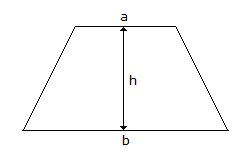A.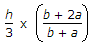B.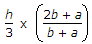C.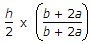D.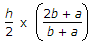Explanation:

No answer description available for this question. Let us discuss.

40.

If the linear velocity of a point on the rim of a wheel of 10 m diameter, is 50 m/sec, its angular velocity will be